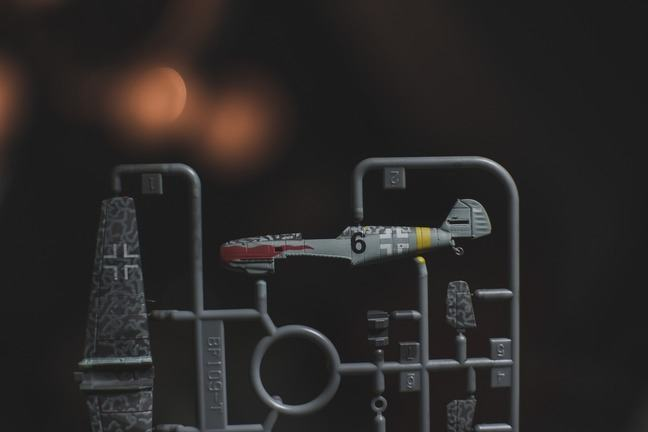## Want to keep learning?

This content is taken from the University of York's online course, Introduction to Cognitive Psychology: An Experimental Science. Join the course to learn more.
3.10

## University of YorkPutting the pieces together

# Thinking visually: Part 1

### Class exercise

We cannot hope to do justice to the length and depth of the debate that has taken place over how best to explain these kinds of data that reflect on visual thinking. But what we can do is get you to engage in some exercises that have featured in the associated literature.

First we provide six simple exercises that tap into your ability to “think visually”.

For each case follow the instructions and write down what the result is.

EXERCISE 1. “Imagine the number ‘7’. Make the diagonal line vertical. Move the horizontal line down to the middle of the vertical line. Now rotate the figure 90 degrees to the left.”

EXERCISE 2. “Imagine the letter ‘B’. Rotate it 90 degrees to the left. Put a triangle directly below it having the same width and pointing down. Remove the horizontal line.”

EXERCISE 3. “Imagine the letter ‘Y’. Put a small circle to the bottom of it. Add a horizontal line halfway up. Now rotate the figure 180 degrees.”

EXERCISE 4. “Imagine the letter ‘K’. Place a square next to it on the left side. Put a circle inside of the square. Now rotate the figure 90 degrees to the left.”

EXERCISE 5. “Imagine a ‘plus’. Add a vertical line on the left side. Rotate the figure 90 degrees to the right. Now remove all lines to the left of the vertical line.”

EXERCISE 6. “Imagine the letter ‘D’. Rotate it 90 degrees to the right. Put the number ‘4’ above it. Now remove the horizontal segment of the ‘4’ to the right of the vertical line.”

Before moving onto the next step, take some time to generate your own answers. The next step provides the answers to these exercises.